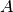Warning

This documents an unmaintained version of NetworkX. Please upgrade to a maintained version and see the current NetworkX documentation.

# from_numpy_matrix¶

from_numpy_matrix(A, parallel_edges=False, create_using=None)[source]

Return a graph from numpy matrix.

The numpy matrix is interpreted as an adjacency matrix for the graph.

Parameters: A (numpy matrix) – An adjacency matrix representation of a graph parallel_edges (Boolean) – If this is True, create_using is a multigraph, and A is an integer matrix, then entry (i, j) in the matrix is interpreted as the number of parallel edges joining vertices i and j in the graph. If it is False, then the entries in the adjacency matrix are interpreted as the weight of a single edge joining the vertices. create_using (NetworkX graph) – Use specified graph for result. The default is Graph()

Notes

If create_using is an instance of networkx.MultiGraph or networkx.MultiDiGraph, parallel_edges is True, and the entries of A are of type int, then this function returns a multigraph (of the same type as create_using) with parallel edges.

If create_using is an undirected multigraph, then only the edges indicated by the upper triangle of the matrixwill be added to the graph.

If the numpy matrix has a single data type for each matrix entry it will be converted to an appropriate Python data type.

If the numpy matrix has a user-specified compound data type the names of the data fields will be used as attribute keys in the resulting NetworkX graph.

Examples

Simple integer weights on edges:

>>> import numpy
>>> A=numpy.matrix([[1, 1], [2, 1]])
>>> G=nx.from_numpy_matrix(A)


If create_using is a multigraph and the matrix has only integer entries, the entries will be interpreted as weighted edges joining the vertices (without creating parallel edges):

>>> import numpy
>>> A = numpy.matrix([[1, 1], [1, 2]])
>>> G = nx.from_numpy_matrix(A, create_using = nx.MultiGraph())
>>> G
{0: {'weight': 2}}


If create_using is a multigraph and the matrix has only integer entries but parallel_edges is True, then the entries will be interpreted as the number of parallel edges joining those two vertices:

>>> import numpy
>>> A = numpy.matrix([[1, 1], [1, 2]])
>>> temp = nx.MultiGraph()
>>> G = nx.from_numpy_matrix(A, parallel_edges = True, create_using = temp)
>>> G
{0: {'weight': 1}, 1: {'weight': 1}}


User defined compound data type on edges:

>>> import numpy
>>> dt = [('weight', float), ('cost', int)]
>>> A = numpy.matrix([[(1.0, 2)]], dtype = dt)
>>> G = nx.from_numpy_matrix(A)
>>> G.edges()
[(0, 0)]
>>> G['cost']
2
>>> G['weight']
1.0